# Catch up on the latest AI articles# Pun-GAN, An AI That Generates Puns!

3 main points
✔️ A task to generate punny sentences using GAN
✔️ Does not require a corpus of punny sentences
✔️ Consists of a punny sentence generator and a word sense discriminator

written by Fuli LuoShunyao LiPengcheng YangLei liBaobao ChangZhifang SuiXu Sun
(Submitted on 24 Oct 2019)

Subjects: Computer Vision and Pattern Recognition (cs.CV); Artificial Intelligence (cs.AI); Machine Learning (cs.LG)code：The images used in this article are either from the paper or created based on it.

## first of all

Generating creatively richer and more interesting text is an important step in building an intelligent natural language generation system. Here, a pun is a clever and funny use of a word that has two meanings (word sense) or a word that has the same sound but different meanings. In this paper, we will focus on the former type of puns. As an example, I will introduce "I used to be a banker but I lost interest" as a pun sentence. The pun word "interest" can be interpreted as both curiosity and profit.

One of the problems of pun generation is that there is no large corpus of puns in which pun sentences are labeled with two-word senses. Early work was rule-based, using templates, which lacked creativity and flexibility. Later work used neural networks for this task, ensuring that the word senses given as input are included in the generated sentence sequences. However, the method was not able to detect whether two-word senses were supported. The detection of punny sentences can be aided by Word Sence Disambiguate (WSD), which aims to identify the correct word sense of a word in a sentence via a multi-class classifier. Based on the above motivation, the proposed method uses Generative Adversarial Net (GAN), a model that takes two words with specific meanings as input to the generator of GAN to generate punny sentences. The discriminator is a model that identifies whether a given sentence is a real sentence or not.

The evaluation of pun generation is also addressed in this paper. In this paper, automatic evaluation and human evaluation were conducted. As a result, we could show that the proposed method, Pun-GAN, generates puns with higher quality in terms of ambiguity and diversity.

## technique

The architecture of GAN is shown in Figure 1, GAN consists of a pun sentence generator $𝐺_𝜃$ and a word sense discriminator $𝐷_𝜙$.

### Generator

The generator $𝐺_𝜃$ outputs a sentence $𝑥$ that not only contains the word $𝑤$ in the sentence but also represents the corresponding two meanings when the two meanings $(𝑠_1, 𝑠_2)$ of the target word 𝑤 are given as input. As a model for the generator, we use the neural constrained language model (2018) of Yu et al. It differs from the traditional neural language model in that it is set up that the word generated at each time step should have the maximum sum of two probabilities computed with $𝑠_1$ and $𝑠_2$ as inputs, respectively. The generation probability of the $t$-th vocabulary is given by: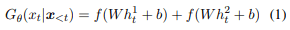Here $h_t^1(h_t^2)$ is the hidden state of the $t$-th step when $s_1(s_2)$ is the input, and $f()$ is the softmax function. $x_{<t}$ is the preceding $t-1$ words. The generation probability of the whole sentence $\bf{x}$ can then be formulated as follows: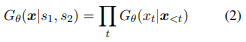### Discriminator

The discriminator classifies classes into $𝑘+1$ categories, which is the number of word senses $𝑘$ plus the "Generative" category (= "Fake" category). The discriminator is an extension of Kageback and Salomonsson's WSD (2016). The discriminator is computed as follows: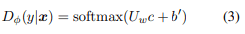where $c$ is the context vector from the bidirectional LSTM when $x$ is the input, $U_w$ is the word-specific parameter, and $y$ is the target label.

### loss function

The training objective of the discriminator is to minimize the following: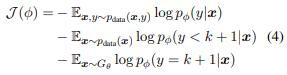Here, $p_{data}$ refers to sentences that have only one-word meaning. To encourage the generation of punny sentences, the discriminator should assign higher rewards to ambiguous punny sentences that can be interpreted as two meanings simultaneously. In the case of punny sentences, the probabilities of $𝐷_𝜙(𝑐_1|𝑥)$ and $𝐷_𝜙(𝑐_2|𝑥)$ are close and the two probabilities should be trained to account for a large number of them. For example, when it is (0.1,0.5,0.4), we should make it more likely to be a punny sentence. On the other hand, when the sentence is (0.1,0.8,0.1), it is assumed to be a general sentence with a meaning (0.8). To achieve this, we design the reward as follows: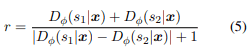The 1 in the denominator is a number to prevent the denominator from being zero.

Minimizing the negative expected reward is the goal when training the generator (Equation 6).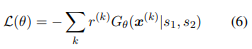The gradient of equation 6 can be approximated as follows: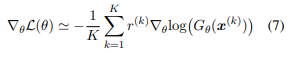## experiment

### Experiment setup

• learning data
• Pre-training of the generator by tagging words in the English Wikipedia corpus with their senses.
• The following three are discriminators
• SemCor, a manually annotated corpus for WSD (first term in Eq. 4)
• Wikipedia corpus (section 2)
• Generated Pun Sentences (Section 3)
• Evaluation Data
• Evaluation Data
• setup
• Randomly initialize Word Embedding with dimension 300
• Sample size K: 32, Learning rate: 0.001, Optimizer: SGD
• Pre-train the generator for 5 epochs and the discriminator for 4 epochs
• In adversarial learning, the generator is trained every 1 epoch and the discriminator is trained every 5 epochs
• baseline model
• LM: Normal RNN
• CLM: A language model that ensures that a given word appears in the generated text.
• CLM+JD: A state-of-the-art model for generating punny sentences by extending CLM.
• assess
• Automatic evaluation: Unusualness is evaluated by subtracting the log probability of the sentence used for training from the log probability of the generated pun sentence, and diversity is evaluated by the ratio of unigram to bigram.
• Human evaluation: A human evaluates 100 randomly sampled outputs on a scale of 1 to 5 based on three criteria.
• Ambiguity: Is the sentence a pun?
• Fluency: How fluent is your writing?
• Overall: Overall rating

### experimental results

The results of the experiments are shown in Tab 1 (automatic evaluation) and Tab 2 (human evaluation): Pun-GAN can generate more creative and unexpected sentences than CLM+JD. However, the results also show that there is still a large gap between the sentences generated by Pun-GAN and the puns written by humans.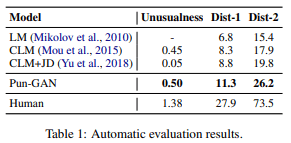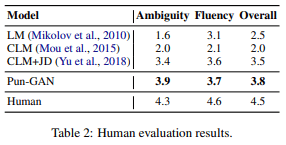An example of a sentence generated by Pun-GAN is shown in Fig. 2.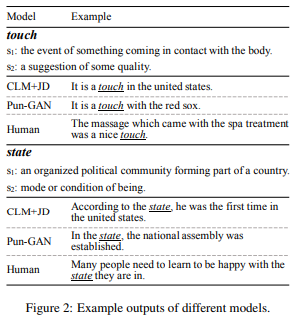Finally, the error types of Pun-GAN are shown in Fig. 3: the error types of Pun-GAN generated sentences were sentences that support only one-word sense (i.e. not punny sentences), uncommon sentences, and sentences with incorrect grammar.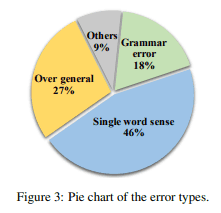## summary

In this article, we introduced a paper that proposed Pun-GAN, an adversarial generation network for generating punny sentences; Pun-GAN consists of a punny sentence generator and a word sense discriminator. In addition, Pun-GAN does not require a pun corpus. Pun-GAN achieves its goal of generating punny sentences by learning via rewards from the discriminator; the authors state that Pun-GAN is generic and flexible and may be extended to other constrained text generation tasks in the future.

If you have any suggestions for improvement of the content of the article,